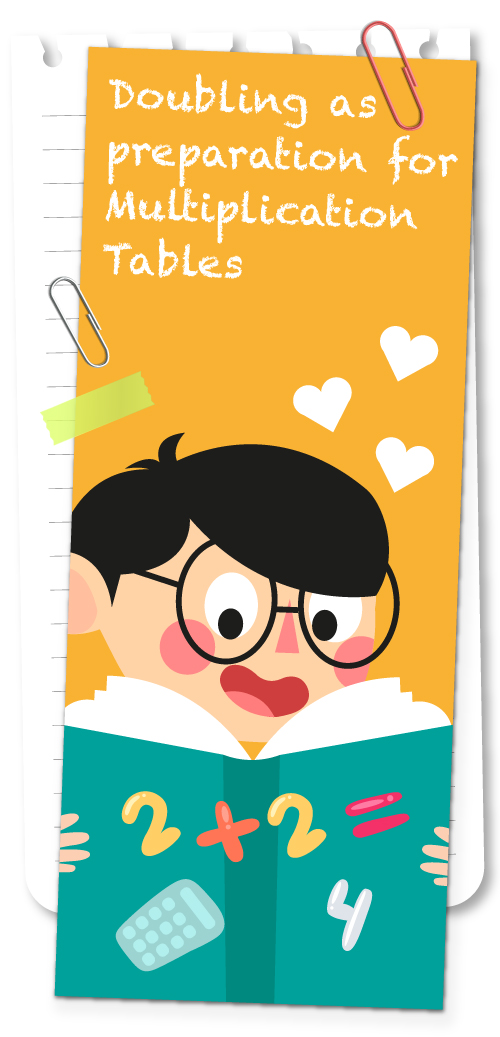### Doubling as Preparation for Multiplication Tables

Many children have difficulty with tables. Although it is an advantage to become 'automatic' with tables it is best if there is understanding and a way of working out the answer by logic rather than just the rote learning that comes from just chanting or singing the table.

Doubling numbers helps to develop this deeper understanding of Tables.

Double two the answer is four
Shoes If there’s two pair of joggers at the door.
2 + 2 = 4
2 X 2 = 4

Double three and you’ll have six
Sometimes you see this in candlesticks.
3 + 3 = 6
2 X 3 = 6

Double four and you’ll have eight
Legs on two cows waiting at a gate.
4 + 4 = 8
2 X 4 = 8

Double five the answer is ten
Players on a court for basketball then.
5 + 5 =10
2 X 5 =10

Double six and twelve you’ll make
Ants legs running on a picnic cake.
6 + 6 = 12
2 X 6 = 12

Double seven is fourteen.
Players on a netball court to be seen.
7 + 7 = 14
2 X 7 = 14

Double eight you’ll have sixteen
Rowers in two boats on the water green
8 + 8 =16
2 X 8 =16

Double nine and eighteen pins there’ll be
In two lanes of a bowling alley.
9 + 9 =18
2 X 9 = 18

Double ten and there’ ll be twenty
Digits on one body and that’s plenty.
ten fingers + ten toes twenty digits but only one nose.
10 + 10 = 20
2 X 10 = 20

Double eleven is twenty two
Players on a hockey field now we’re nearly through.
11 + 11 = 22
2 X 11 = 22

Double twelve is twenty four
Hours in a day and there can’t be more.
12 + 12 = 24
2 X 12 = 24Help children to internalise the concepts by drawing the appropriate pictures and then counting the appropriate parts.

Once the basic doubling of numbers is well known proceed to doubling other figures of two numbers.

Start by using numbers where the units number is under 5.

Double the tens number first then the units number

eg 14 doubled is 28
24 doubled is 48
42 doubled is 84

Now try numbers where the units number is 5 or greater.
Which means there will be an extra ‘ten’ to add to the tens side.
Have the child first check if there WILL be an extra ten.

Double 17 Will there be an extra ten? (YES) because 7 = 7 is 14
So one ten for each 17 plus an extra ten will mean three tens and the four from the fourteen

Double 17 = 34
Double 27 = 54
Double 37 = 74
Double 47 = 94

Double numbers where the tens number is more than 5 and show there will be a hundred.

Double 53 =106
Double 63 = 126
Double 73 = 146
Double 83 = 166